Ch 1. Particle General Motion Multimedia Engineering Dynamics Position,Vel & Accel. Accel. varyw/ Time Accel. Constant Rect. Coordinates Norm/Tang. Coordinates Polar Coordinates RelativeMotion
 Chapter - Particle - 1. General Motion 2. Force & Accel. 3. Energy 4. Momentum - Rigid Body - 5. General Motion 6. Force & Accel. 7. Energy 8. Momentum 9. 3-D Motion 10. Vibrations Appendix Basic Math Units Basic Equations Sections Search eBooks Dynamics Fluids Math Mechanics Statics Thermodynamics Author(s): Kurt Gramoll ©Kurt GramollDYNAMICS - THEORY

If an object moves in a curved path, it is said to be in curvilinear motion. It is useful to use vectors to describe the motion of a curved path.

Position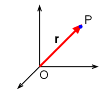Position Vector

To define the position of a point P, select a fixed coordinate system with an origin O. The position of the point P is defined by a position vector, r, connecting O to P.

If P is in motion relative to O, then the position vector will change with time,
 r = r(t)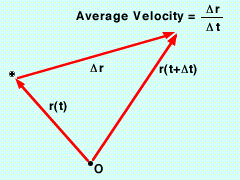Position and Velocity
Average Velocity

Here, r(t) denotes the position of P at time t. If r(t + Δt) denotes the position at time t + Δt, then Δr represents the change in position,

Δr = r(t + Δt) - r(t)

The average velocity is the overall change of position over the overall change of time,

Average velocity = Δr / Δt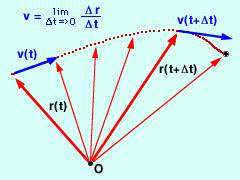Instantaneous Velocity
Instantaneous Velocity

Usually, instantaneous velocity is needed, not average velocity. To determine the instantaneous velocity, take the average velocity over smaller and smaller increments of time,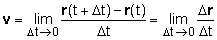v = dr/dt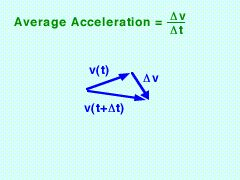Acceleration
Acceleration

If v(t) denotes the velocity at time t and v(t + Δt) denotes the velocity at time t + Δt, then

Δv = v(t + Δt) - v(t)

Average acceleration = Δv/Δt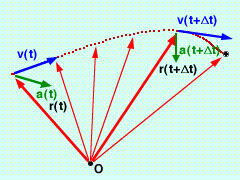Instantaneous Acceleration
Instantaneous Acceleration

To determine the instantaneous acceleration, take the average acceleration over smaller and smaller increments of time, giving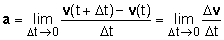a = dv/dt

 a = dv/dt

Rectangular Coordinates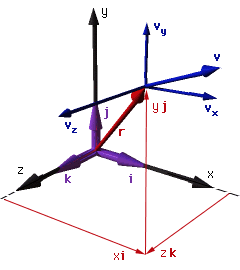Rectangular Coordinate System

Let the origin O reside at the origin of the Cartesian axis. Resolve the position vector r(t) into rectangular x, y, and z components,

r = xi + yj + zk

Velocity

Differentiate to find velocity, giving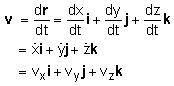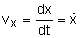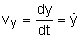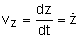Acceleration

To find the acceleration, take a derivative of the velocity, which gives,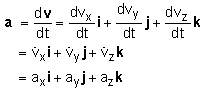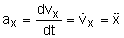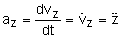Rectangular motion is useful if ax depends only on t, x, and/or vx (likewise for ay and az).

Note that the equations describing the motion in each coordinate direction are identical to those of linear motion, as denoted in the Position, Velocity, Acceleration section.

2-D Projectile Problems

Most problems involve projectiles with constant gravity acceleration in only the y direction. In this special case, the rectilinear motion equations become (assumes up is positive y),

 y direction (gravity) x direction (no gravity)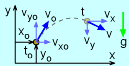vy(t) = vyo - g (t - to) vx(t) = vxo y(t) = yo + vyo (t - to) - g (t - to)2 / 2 x(t) = xo + vxo (t - to) vy2 = vo2 - 2 g (x - xo) vx2 = vxo2

Practice Homework and Test problems now available in the 'Eng Dynamics' mobile app
Includes over 400 problems with complete detailed solutions.
Available now at the Google Play Store and Apple App Store.